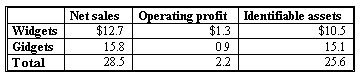### CFA Practice Question

There are 534 practice questions for this study session.

### CFA Practice Question

ABC Co. has the following segment reporting information for 2015.Gidgets have a return on assets of ______.
A. 6.0%
B. 10.2%
C. 104.6%

User Comment
PedroEdmundo ROA=NI/ASSET
Xocrevilo Is net income provided in this question?!
Kuki Net income would be your operating profit.

ROA = Op Profit / Identifiable Asset = 0.9 / 15.1
= 5.96% approx 6%
brandsat Operating profit is before taxes, NI would be lower, so only logical answer is 5.7 %.

Why not ??
Smiley225 Good logic brandsat, BUT difficult to assume what tax rate to apply as it is not given.
Lamkerst ROA=EBIT/Assets, not NI/Assets.
Assets (donominator) belong to all security holders, so numerator should also incorporate returns for all the security holders, not only equity holders. Otherwise, it is inconsistent.
gill15 Return on Assets can be calculated many ways...not just one...here because NI is not given you use operating ROA = OI/Avg Assets.
Oksanata the key word here is "segment" . Segment ROA = Segment profit(loss)/segment asset. Such ROA measures the OPERATING income generated per the level of segment assets (investment).